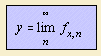# (parabola) bifurcation

## fractal

last updated: 2003-08-13where f x,n is an attractor

For an infinite recurrent parabola y = ax (1-x) we can compute the possible function values, as function of the parameter a. The resulting pattern is a bifurcation 1), a forklike ramification. The shown bifurcation belongs to the above mentioned parabola. For other polynomials sometimes symmetry around the x-axis be reached.
Mind the vertical white bands and the black arcs in the chaotic part at the right side of the picture. These are regions where some values are impossible (white) or very popular (black).Special situations on the curve are:

• a=0 and a=1 are on the curve
• at a=3 the curve is split in two branches
• at a= 1 +6 the curve is split from two to four branches

The generalization for the bifurcation to the complex numbers is the Mandelbrot set.

notes

1) furca (Lat.) = fork, bis (Lat.) = twice